# IB DP Physics: SL复习笔记2.1.8 Terminal Speed

### Fluid Resistance & Terminal Speed

#### Fluid Resistance

• When an object moves through a fluid (a gas or a liquid) then there are resistive forces for that movement.
• These forces are known as drag forces
• Examples of drag forces are friction and air resistance
• Drags forces:
• Are always in the opposite direction to the motion of the object
• Never speed an object up or start them moving
• Slow down an object or keep them moving at a constant speed
• Convert kinetic energy into heat and sound
• Lift is an upwards force on an object moving through a fluid. It is perpendicular to the fluid flow
• For example, as an aeroplane moves through the air, it pushes down on the air to change its direction
• This causes an equal and opposite reaction upwards on the wings (lift) due to Newton's third law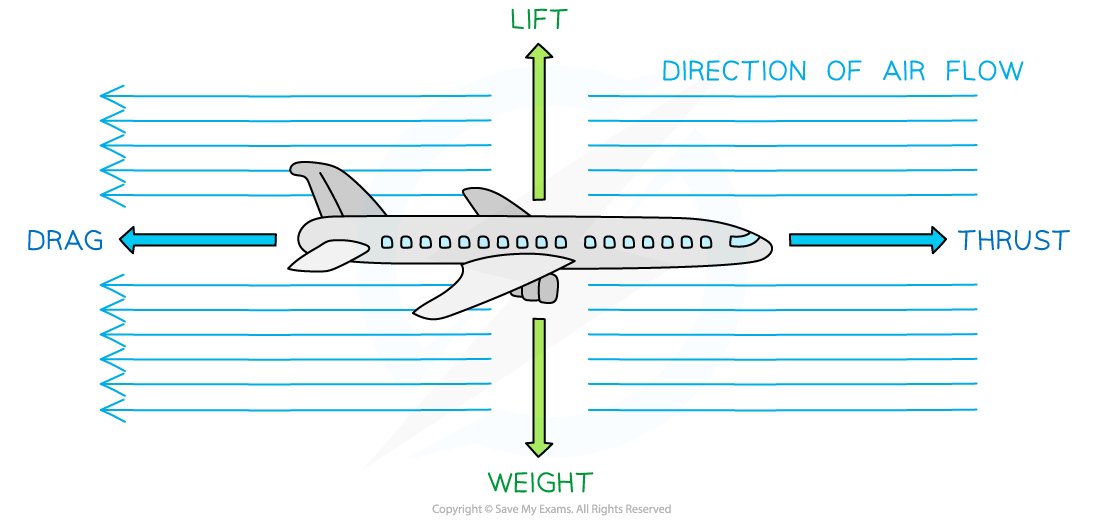Drag forces are always in the opposite direction to the thrust (direction of motion). Lift is always in the opposite direction to the weight

• A key component of drag forces is it increases with the speed of the object
• This is shown in the diagram below: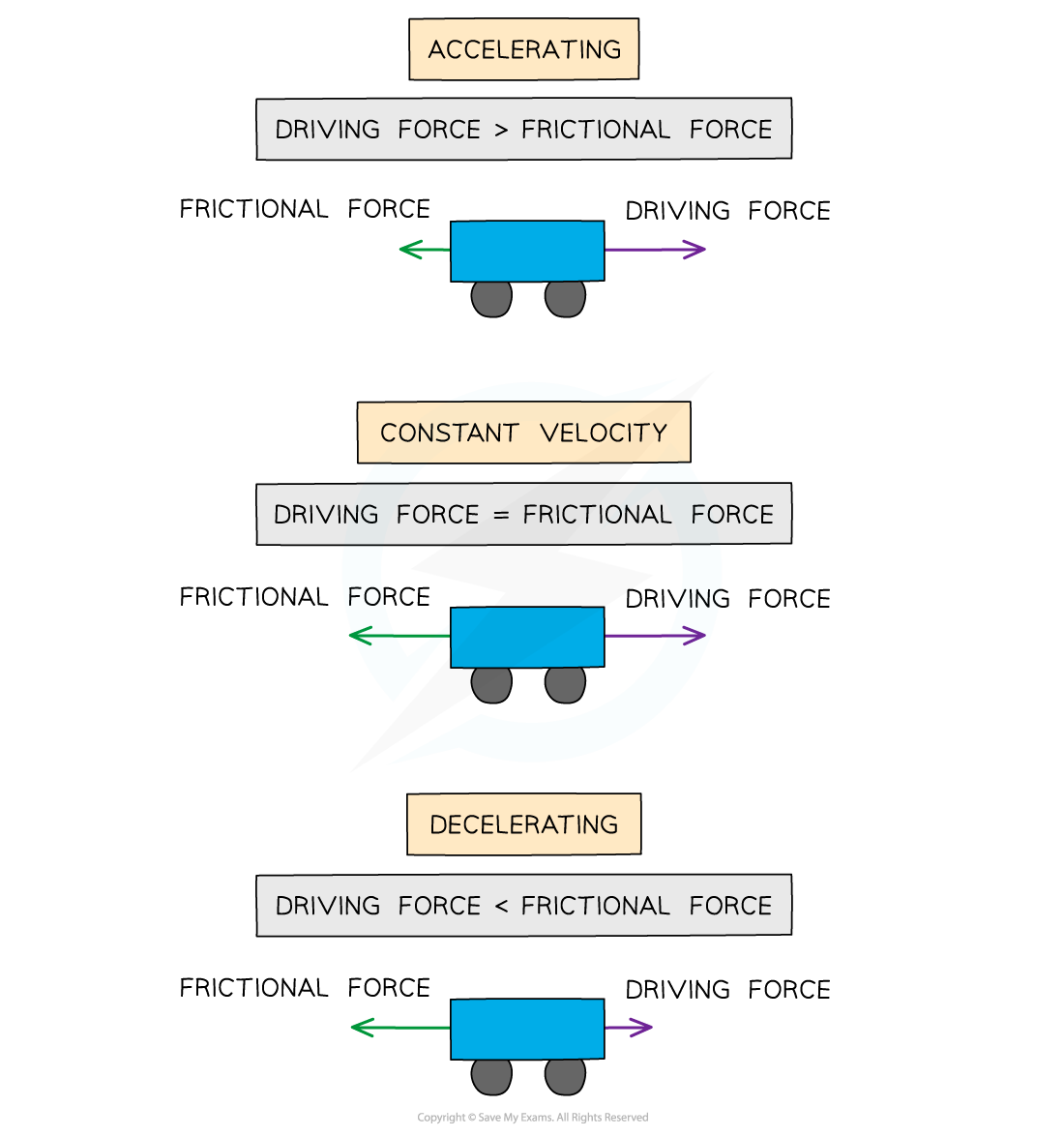Frictional forces on a car increase with speed

#### Air Resistance & Projectile Motion

• Air resistance decreases the horizontal component of the velocity of a projectile
• This means both its range and maximum height is decreased compared to no air resistanceA projectile with air resistance travels a smaller distance and has a lower maximum height than one without air resistance

• The angle and speed of release of a projectile is varied to produce either a longer flight path or cover a larger distance, depending on the situation
• For sports such as the long jump or javelin, an optimum angle against air resistance is used to produce the greatest distance
• For gymnastics or a ski jumper, the initial vertical velocity is made as large as possible to reach a greater height and longer flight path

#### Terminal Velocity

• For a body in free fall, the only force acting is its weight and its acceleration g is only due to gravity.
• The drag force increases as the body accelerates
• This increase in velocity means the drag force also increases
• Due to Newton’s Second Law, this means the resultant force and therefore acceleration decreases (recall F = ma)
• When the drag force is equal to the gravitational pull on the body, the body will no longer accelerate and will fall at a constant velocity
• This velocity is called the terminal velocity
• Terminal velocity can occur for objects falling through a gas or a liquid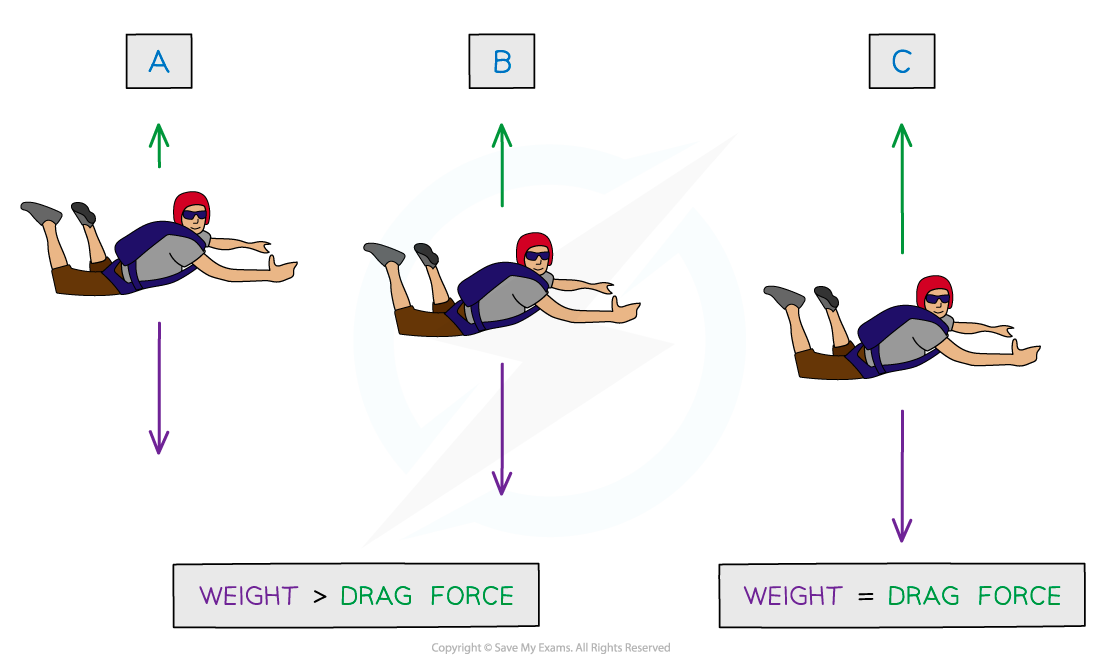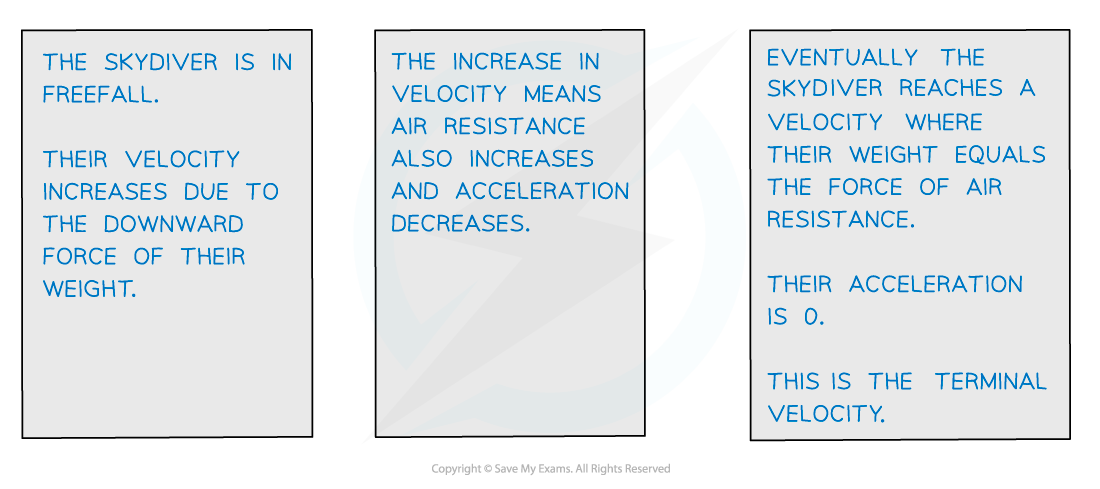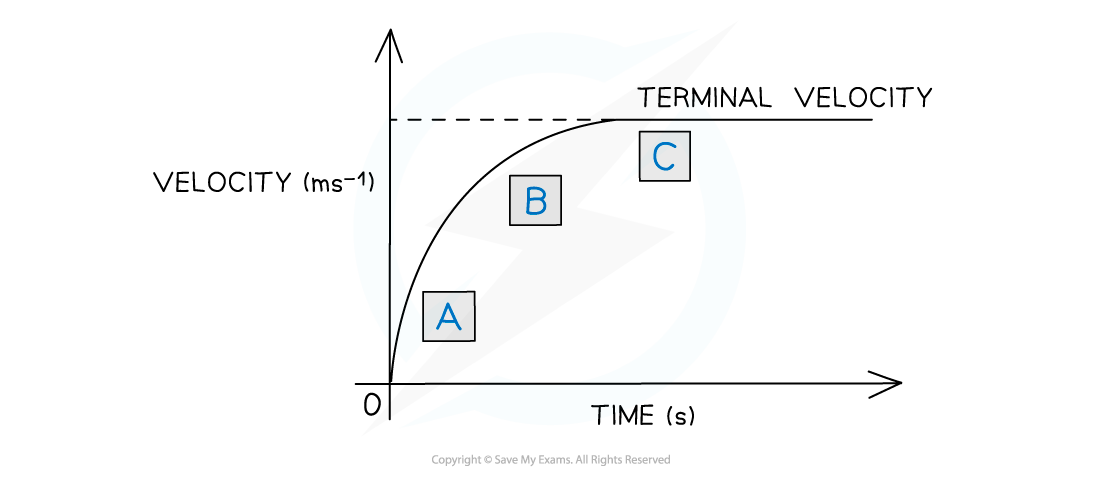A skydiver in freefall reaching terminal velocity

• The graph shows how the velocity of the skydiver varies with time
• Since the acceleration is equal to the gradient of a velocity-time graph, the acceleration decreases and eventually becomes zero when terminal velocity is reached
• After the skydiver deploys their parachute, they decelerate to a lower terminal velocity to reduce the impact on landing
• This is demonstrated by the graph below: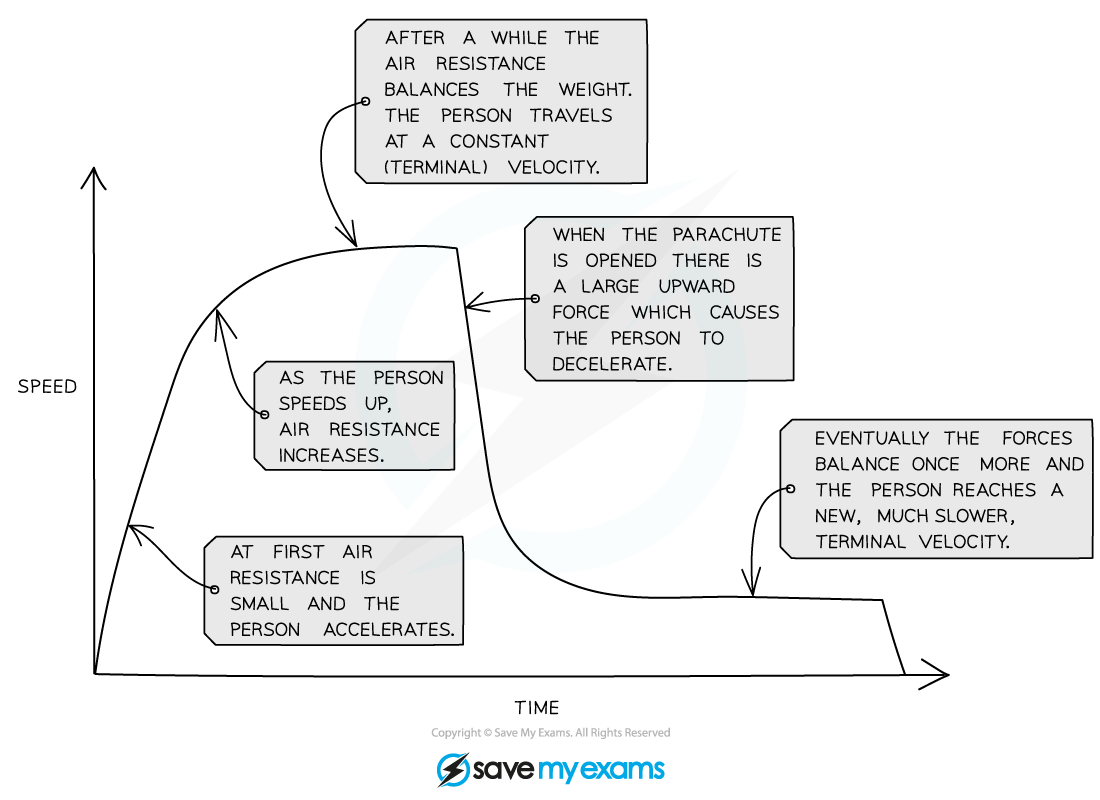A graph showing the changes in speed of the skydiver throughout their entire journey in freefall

#### Worked Example

Skydivers jump out of a plane at intervals of a few seconds.

Skydivers A and B want to join up as they fall.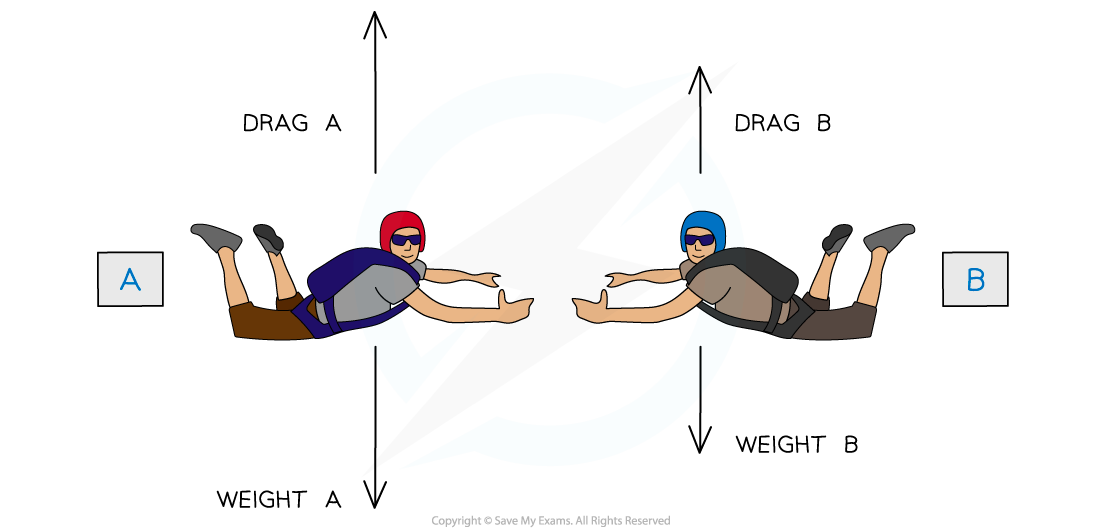If A is heavier than B, who should jump first?

• Skydiver B should jump first since he will take longer to reach terminal velocity
• This is because skydiver A has a higher mass, and hence, weight
• More weight means higher acceleration and hence higher speed, therefore, A will reach terminal velocity faster than B

#### Exam Tip

A common misconception is that skydivers move upwards when their parachutes are deployed - however, this is not the case, they are in fact decelerating to a lower terminal velocityIf a question considers air resistance to be ‘negligible’ this means in that question, air resistance is taken to be so small it will not make a difference to the motion of the body. You can take this to mean there are no drag forces acting on the body.